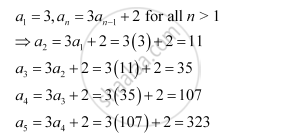CBSE (Arts) Class 11CBSE
Share
Notifications

View all notifications
Books Shortlist
Your shortlist is empty

# Write the First Five Terms of the Following Sequence and Obtain the Corresponding Series: A_1 = 3, A_N = 3a_( N-1) + 2 for All N > 1 - CBSE (Arts) Class 11 - Mathematics

Login
Create free account

Forgot password?

#### Question

Write the first five terms of the following sequence and obtain the corresponding series:

a_1 = 3, a_n = 3a_( n-1) + 2 for all n > 1

#### SolutionHence, the first five terms of the sequence are 3, 11, 35, 107, and 323.

The corresponding series is 3 + 11 + 35 + 107 + 323 + …

Is there an error in this question or solution?

#### APPEARS IN

NCERT Solution for Mathematics Textbook for Class 11 (2018 to Current)
Chapter 9: Sequences and Series
Q: 11 | Page no. 181
Solution Write the First Five Terms of the Following Sequence and Obtain the Corresponding Series: A_1 = 3, A_N = 3a_( N-1) + 2 for All N > 1 Concept: Concept of Series.
S